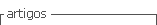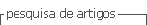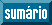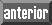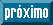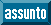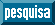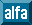## Artigo

•Citado por SciELO
•Acessos

•Citado por Google
•Similares em SciELO
•Similares em Google

## versão impressa ISSN 0012-7353

#### Resumo

ORTIZ-YANEZ, Jesús Francisco  e  PINA-MONARREZ, Manuel Román. Discrimination between the lognormal and Weibull distributions by using multiple linear regression. Dyna rev.fac.nac.minas [online]. 2018, vol.85, n.205, pp.9-18. ISSN 0012-7353.  http://dx.doi.org/10.15446/dyna.v85n205.66658.

In reliability analysis, both the Weibull and the lognormal distributions are analyzed by using the observed data logarithms. While the Weibull data logarithm presents skewness, the lognormal data logarithm is symmetrical. This paper presents a method to discriminate between both distributions based on: 1) the coefficients of variation (CV), 2) the standard deviation of the data logarithms, 3) the percentile position of the mean of the data logarithm and 4) the cumulated logarithm dispersion before and after the mean. The efficiency of the proposed method is based on the fact that the ratio of the lognormal ( b1ln ) and Weibull ( b1w ) regression coefficients (slopes) b1ln/b1w efficiently represents the skew behavior. Thus, since the ratio of the lognormal ( Rln ) and Weibull ( Rw ) correlation coefficients Rln/Rw (for a fixed sample size) depends only on the b1ln/b1w ratio, then the multiple correlation coefficient R2 is used as the index to discriminate between both distributions. An application and the impact that a wrong selection has on R(t) are given also.

Palavras-chave : Weibull distribution; lognormal distribution; discrimination process; multiple linear regression; Gumbel distribution.

· resumo em Espanhol     · texto em Inglês     · Inglês (pdf )